## How to Convert Square Miles to Acres | Area Units

The image below represents square miles to acres.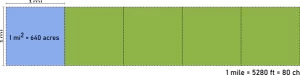To convert square miles to acres, one essential parameter is needed and this parameter is Unit Value.

The formula for converting square miles to acres:

1 Square Mile = 640 Acres

Let’s solve an example;
Find the conversion of square miles to acres when the unit value is 4.

1 Square Mile = 640 Acres

Then,

4 Square Miles = (4 x 640) Acres
4 Square Miles = 2560 Acres

Therefore, the acre(s) is 2560.

## How to Convert Square Miles to Square Yards | Area Units

The image below represents square miles to square yards.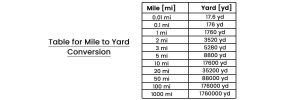To convert square miles to square yards, one essential parameter is needed and this parameter is Unit Value.

The formula for converting square miles to square yards:

1 Square Mile = 3097600 Square Yards

Let’s solve an example;
Find the conversion of square miles to square yards when the unit value is 30.

1 Square Mile = 3097600 Square Yards

Then,

30 Square Miles = (30 x 3097600) Square Yards
30 Square Miles = 92928000 Square Yards

Therefore, the square yard(s) is 92928000.

## How to Convert Square Miles to Square Feet | Area Units

The image below represents square miles to square feet.To convert square miles to square feet, one essential parameter is needed and this parameter is Unit Value.

The formula for converting square miles to square feet:

1 Square Mile = 27878400 Square Feet

Let’s solve an example;
Find the conversion of square miles to square feet when the unit value is 22.

1 Square Mile = 27878400 Square Feet

Then,

22 Square Miles = (22 x 27878400) Square Feet
22 Square Miles = 613324800 Square Feet

Therefore, the square feet is 613324800.

## How to Convert Square Miles to Square Inches | Area Units

The image below represents square miles to square inches.To convert square miles to square inches, one essential parameter is needed and this parameter is Unit Value.

The formula for converting square miles to square inches:

1 Square Mile = 401489600 Square Inches

Let’s solve an example;
Find the conversion of square miles to square inches when the unit value is 14.

1 Square Mile = 401489600 Square Inches

Then,

14 Square Miles = (14 x 401489600) Square Inches
14 Square Miles = 56202854400 Square Inches

Therefore, the square inch(es) is 56202854400.

## How to Convert Square Miles to Square Kilometers | Area Units

The image below represents square miles to square kilometers.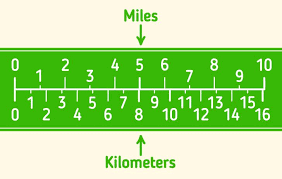To convert square miles to square kilometers, one essential parameter is needed and this parameter is Unit Value.

The formula for converting square miles to square kilometers:

1 Square Mile = 2.589988 Square Kilometers

Let’s solve an example;
Find the conversion of square miles to square kilometers when the unit value is 8.

1 Square Mile = 2.589988 Square Kilometers

Then,

8 Square Miles = (8 x 2.589988) Square Kilometers
8 Square Miles = 20.719904 Square Kilometers

Therefore, the square kilometer(s) is 20.719.

## How to Convert Square Miles to Hectares | Area Units

The image below represents square miles to hectares.To convert square miles to hectares, one essential parameter is needed and this parameter is Unit Value.

The formula for converting square miles to hectares:

1 Square Mile = 258.9988 Hectares

Let’s solve an example;
Find the conversion of square miles to hectares when the unit value is 4.

1 Square Mile = 258.9988 Hectares

Then,

4 Square Miles = (4 x 258.9988) Hectares
4 Square Miles = 1035.9952 Hectares

Therefore, the hectare(s) is 1035.99.

## How to Convert Square Miles to Square Meters | Area Units

The image below represents square miles to square meters.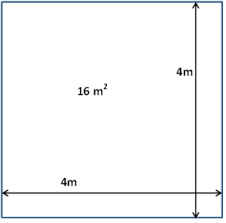To convert square miles to square meters, one essential parameter is needed and this parameter is Unit Value.

The formula for converting square miles to square meters:

1 Square Mile = 2589988 Square Meters

Let’s solve an example;
Find the conversion of square miles to square meters when the unit value is 3.

1 Square Mile = 2589988 Square Meters

Then,

3 Square Miles = (3 x 2589988) Square Meters
3 Square Miles = 7769964 Square Meters

Therefore, the square meter(s) is 7769964.

## How to Convert Square Miles to Square Centimeters | Area Units

The image below represents square miles to square centimeters.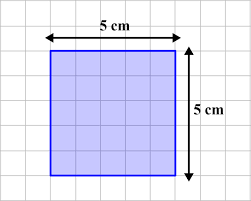To convert square miles to square centimeters, one essential parameter is needed and this parameter is Unit Value.

The formula for converting square miles to square centimeters:

1 Square Mile = 25899881103 Square Centimeters

Let’s solve an example;
Find the conversion of square miles to square centimeters when the unit value is 2.

1 Square Mile = 25899881103 Square Centimeters

Then,

2 Square Miles = (2 x 25899881103) Square Centimeters
2 Square Miles = 51799762206 Square Centimeters

Therefore, the square centimeter(s) is 51799762206.

## How to Convert Square Miles to Square Millimeters | Area Units

The image below represents square miles to square millimeters.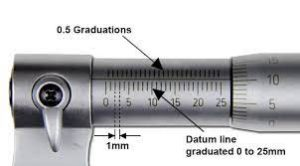To convert square miles to square millimeters, one essential parameter is needed and this parameter is Unit Value.

The formula for converting square miles to square millimeters:

Let’s solve an example;
Find the conversion of square miles to square millimeters when the unit value is 6.

1 Square Mile = 2589988110336 Square Millimeters

Then,

6 Square Miles = (6 x 2589988110336) Square Millimeters
6 Square Miles = 15539928662016 Square Millimeters

Therefore, the square millimeter(s) is 15539928662016.

## How to Convert Square Miles to Square Micrometer | Area Units

The image below represents square miles to square micrometers.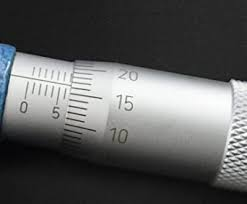To convert square miles to square micrometers, one essential parameter is needed and this parameter is Unit Value.

The formula for converting square miles to square micrometers:

1 Square Mile = 2589990000000000000 Square Micrometers

Let’s solve an example;
Find the conversion of square miles to square micrometers when the unit value is 5.

1 Square Mile = 2589990000000000000 Square Micrometers

Then,

5 Square Miles = (5 x 2589990000000000000) Square Micrometers
5 Square Miles = 12949950000000000000 Square Micrometers

Therefore, the square micrometer(s) is 12949950000000000000.